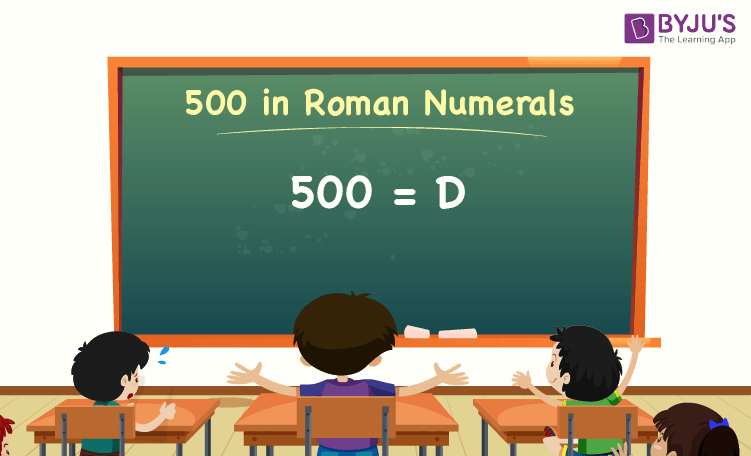# 500 in Roman Numerals

The number 500 in Roman numerals is D. We know that the system of expressing numbers using Roman alphabets is known as Roman numerals. In this article, let us discuss how to write the number 500 in Roman numerals, with many solved examples in detail.

 500 in Roman Numerals = D

## How to Write 500 in Roman Numerals?To write the number 500 in Roman numerals, we can directly denote 500 with the Roman numeral “D”. There is no such expandable method to write 500 in Roman numerals, where we can perform the addition or subtraction operation.

Hence, 500 in Roman numeral is D.

### Roman Numerals Related to the Number 500

Some of the Roman numerals related to the number 500 are as follows:

Roman Numeral for 501 = 500 + 1 = D + I = DI

Roman Numeral for 502 = 500 + 2 = D + I + I = DII

Roman Numeral for 503 = 500 + 3 = D + I + I + I = DIII

Roman Numeral for 504 = 500 + 4 = D + IV = DIV

Roman Numeral for 505 = 500 + 5 = D + V = DV

Roman Numeral for 506 = 500 + 6 = D + V + I = DVI

Roman Numeral for 507 = 500 + 7 = D + V + I + I = DVII

Roman Numeral for 508 = 500 + 8 = D + V + I + I + I = DVIII

Roman Numeral for 509 = 500 + 9 = D + IX = DIX

Roman Numeral for 510 = 500 + 10 = D + X = DX

## Video Lesson on Roman Numerals### 500 in Roman Numerals – Solved Examples

Example 1:

What is the value of 1000 – 500? Express the value in Roman numerals.

Solution:

The value of 1000 – 500 is 500.

Thus, 500 in Roman numerals is D.

Example 2:

Find the sum of D and XI. Express the sum in Roman numerals.

Solution:

We know that,

D = 500

XI = 11

So, D + XI = 500 + 11 = 511.

Hence, 511 in Roman numerals is DXI.

Stay tuned to BYJU’S – The Learning App, and download the app today to watch many engaging videos.

## Frequently Asked Questions on 500 in Roman Numerals

Q1

### What is 500 in Roman numerals?

500 in Roman numerals is D.

Q2

### Express the value of 550 – 50 in Roman numerals.

The value of 550 – 50 is 500. Hence, 500 in Roman numerals is D.

Q3

### What should be added to 400 to get the Roman numerals D. Express the value in words.

100 should be added to 400 to get the Roman numerals D, which is 500. 100 in Roman numerals is C.